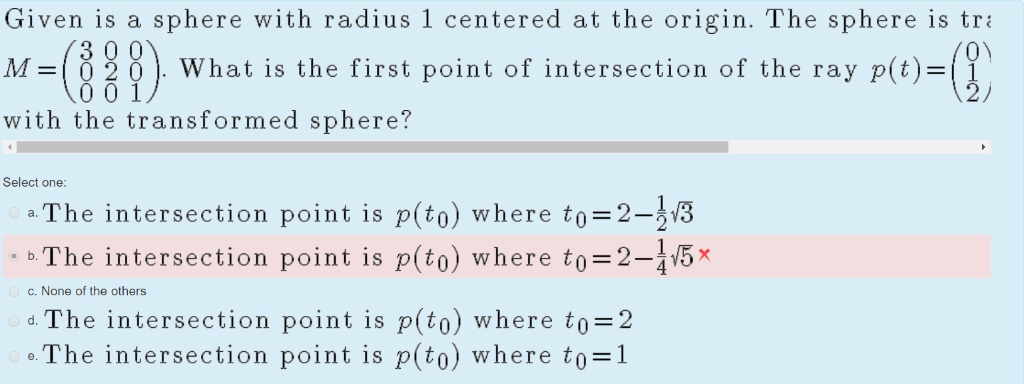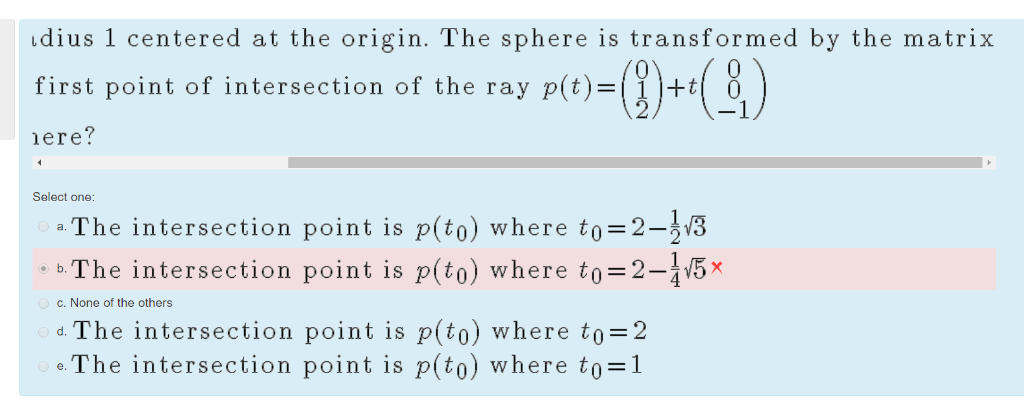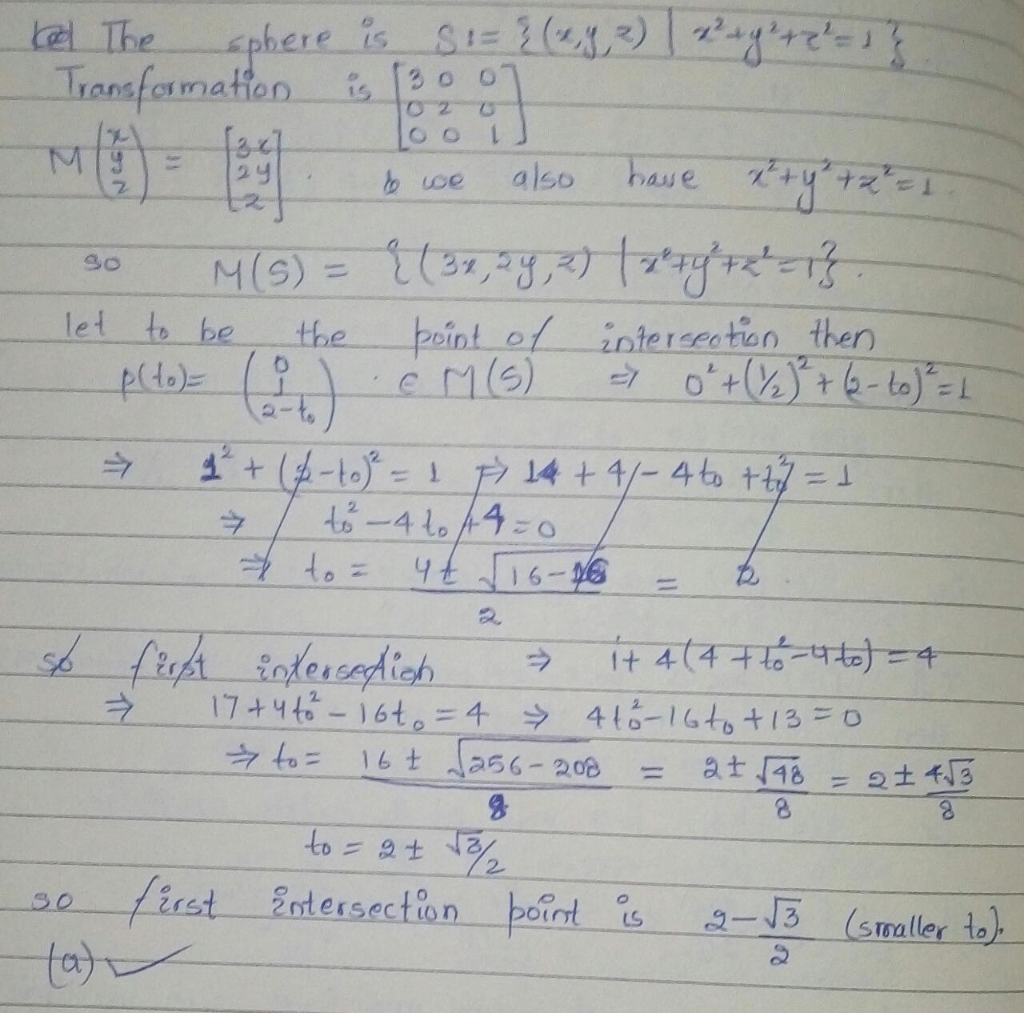# sphere with radius 1 centered at the origin. The sphere is tr Given is a 3 0 0 =10 2 0O 0 0 1 O) What is the first poin...sphere with radius 1 centered at the origin. The sphere is tr Given is a 3 0 0 =10 2 0O 0 0 1 O) What is the first point of intersection of the ray p(t) 2/ |M with the transformed sphere? Select one: The intersection point is p(to) where to=2-v3 o b.The intersection point is p(to) where to=2-v5x a c. None of the others The intersection point is p(to) where to=2 o. The intersection point is p(to) where to=1
dius 1 centered at the origin. The sphere is transformed by the matrix p(t)= first point of intersection of the ray ere? Select one: a. The intersection point is p(to) where to=2-3v3 b.The intersection point is p(to) where to=2-15x c. None of the others d. The intersection point is p(to) where to=2 e. The intersection point is p(to) where to=1please rate

##### Add Answer of: sphere with radius 1 centered at the origin. The sphere is tr Given is a 3 0 0 =10 2 0O 0 0 1 O) What is the first poin...
More Homework Help Questions Additional questions in this topic.